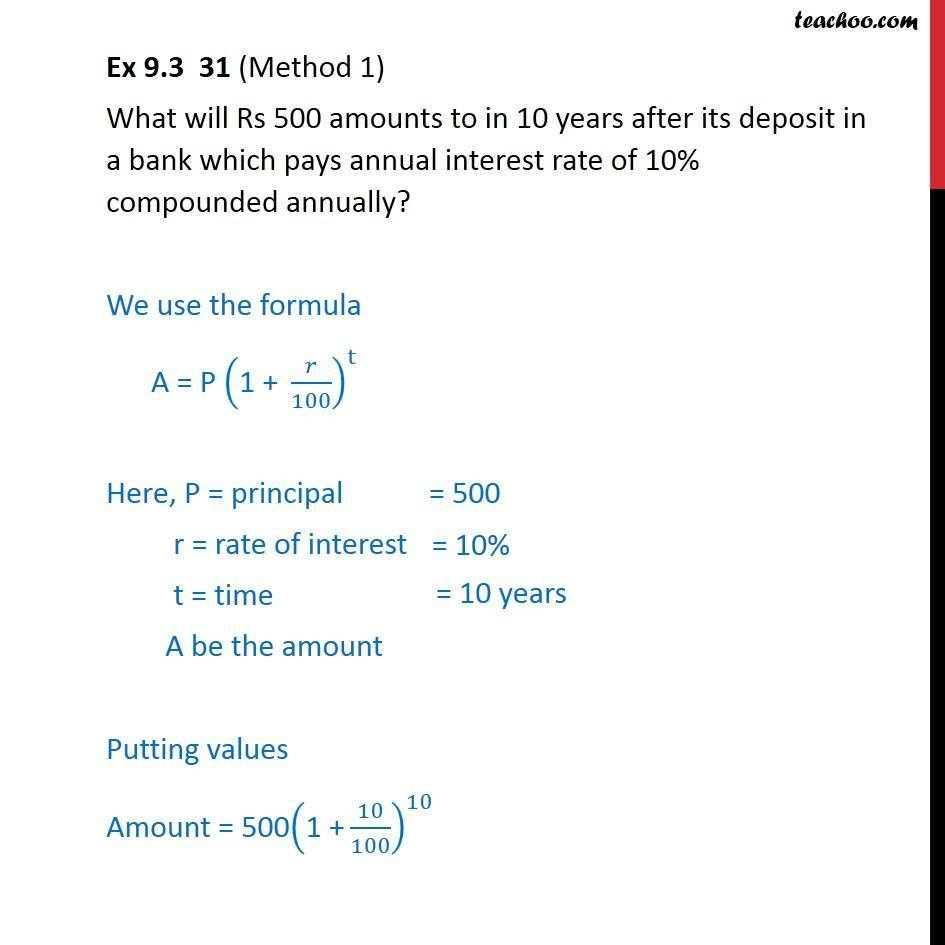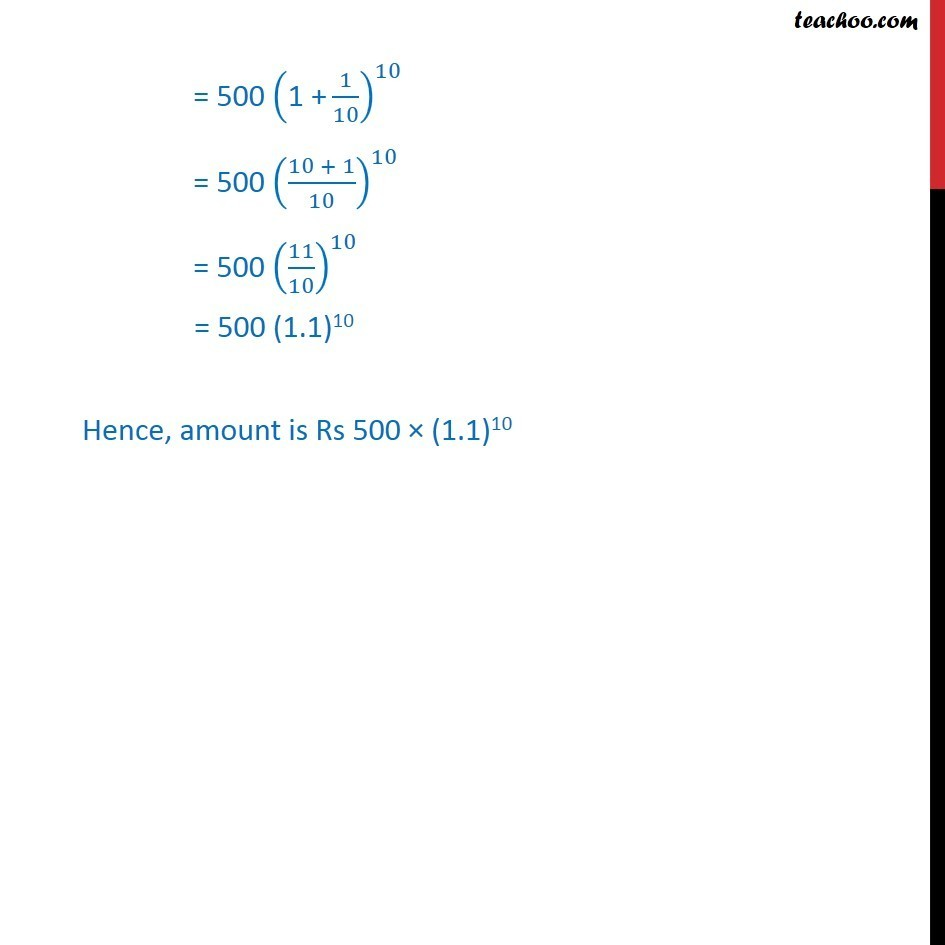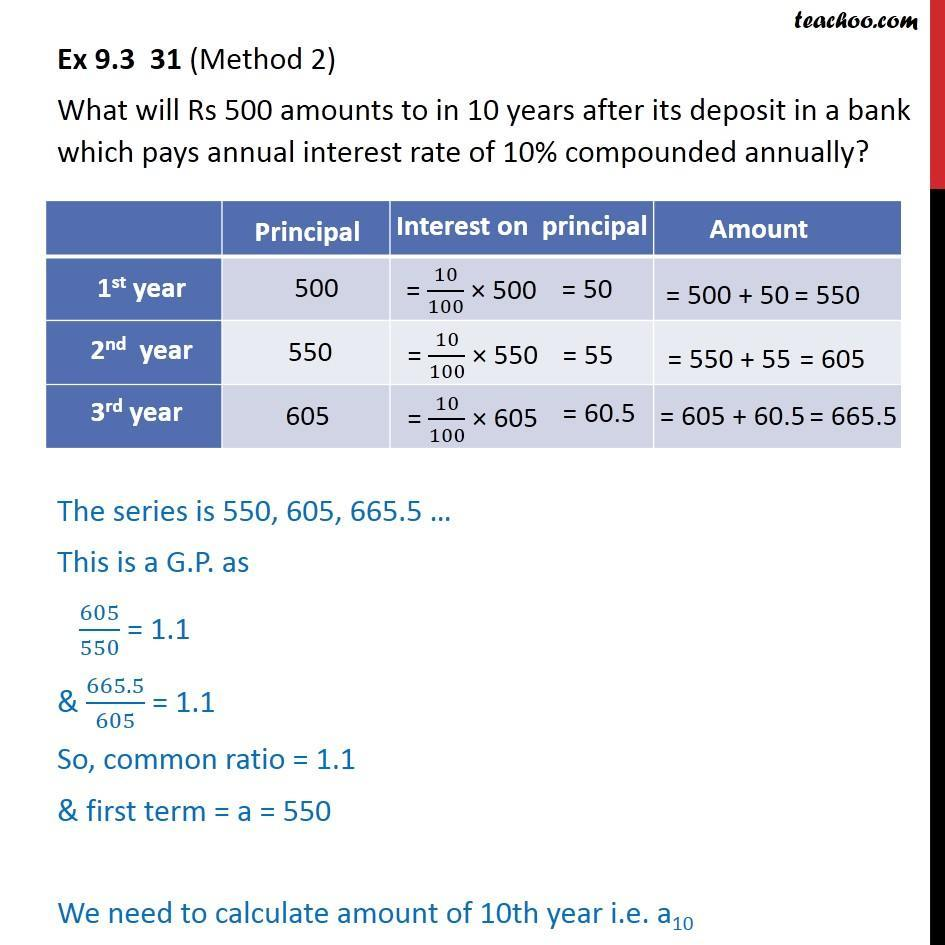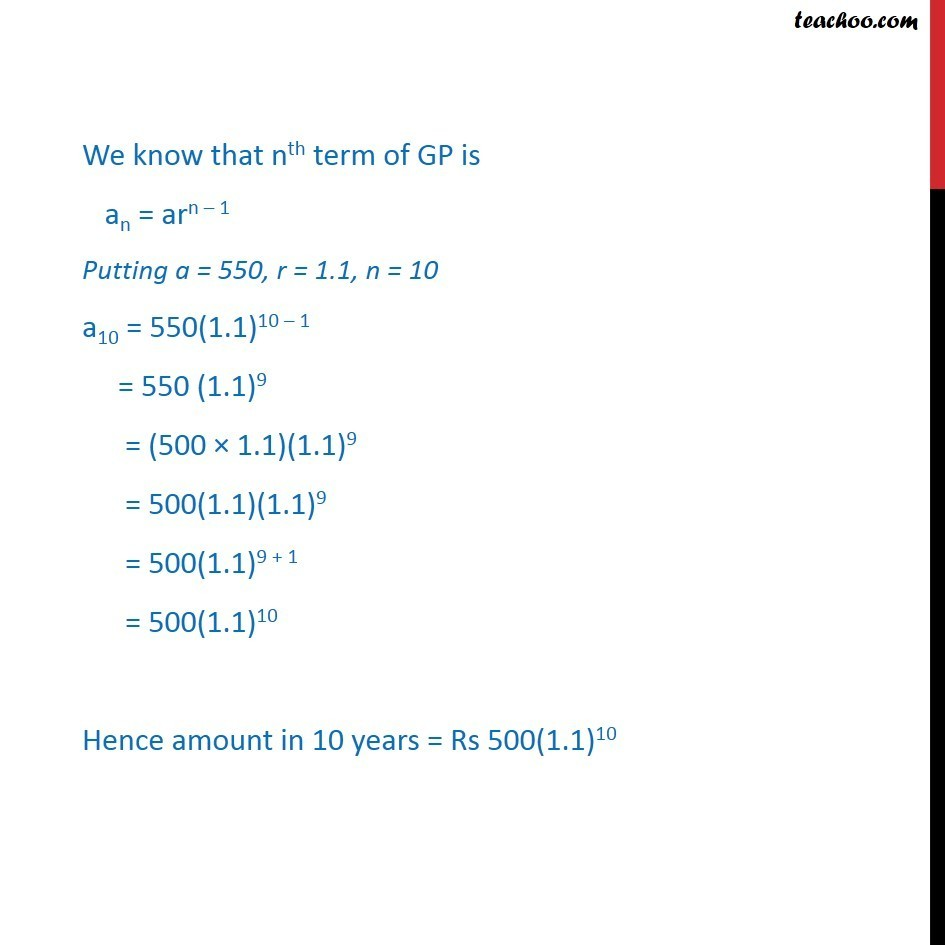Ex 8.2

Chapter 8 Class 11 Sequences and Series
Serial order wiseLearn in your speed, with individual attention - Teachoo Maths 1-on-1 Class

### Transcript

Ex 8.2 31 (Method 1) What will Rs 500 amounts to in 10 years after its deposit in a bank which pays annual interest rate of 10% compounded annually? We use the formula A = P ("1 + " 𝑟/100)^t Here, P = principal r = rate of interest t = time A be the amount Putting values Amount = 500("1 +" 10/100)^10 = 500 ("1 +" 1/10)^10 = 500 ((10 + 1)/10)^10 = 500 (11/10)^10 = 500 (1.1)10 Hence, amount is Rs 500 × (1.1)10 Ex 8.2 31 (Method 2) What will Rs 500 amounts to in 10 years after its deposit in a bank which pays annual interest rate of 10% compounded annually? The series is 550, 605, 665.5 … This is a G.P. as 605/550 = 1.1 & 665.5/605 = 1.1 So, common ratio = 1.1 & first term = a = 550 We need to calculate amount of 10th year i.e. a10 We know that nth term of GP is an = arn – 1 Putting a = 550, r = 1.1, n = 10 a10 = 550(1.1)10 – 1 = 550 (1.1)9 = (500 × 1.1)(1.1)9 = 500(1.1)(1.1)9 = 500(1.1)9 + 1 = 500(1.1)10 Hence amount in 10 years = Rs 500(1.1)10